# LOG#061. SM (III): EW sector.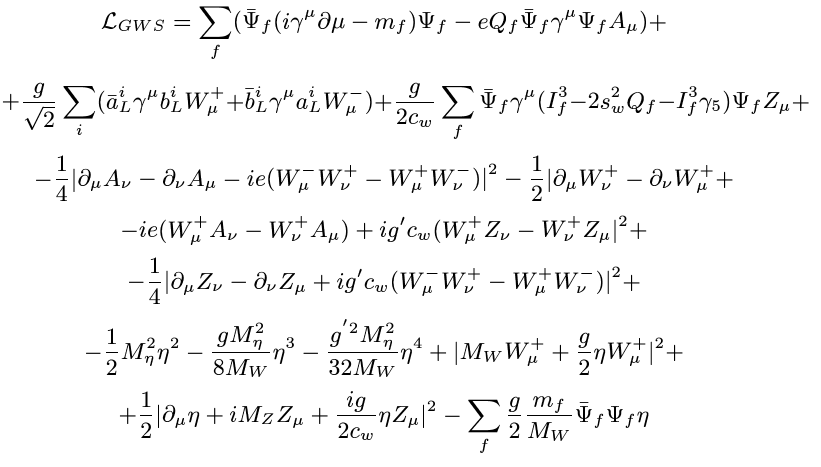The above picture is the EW lagrangian! Is it simple? Is it beautiful? It depends on your taste! It is called the GSW (Glashow-Salam-Weinberg) lagrangian.

The electroweak (EW) part/theory is based on the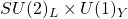gauge symmetry group. Before spontaneous symmetry breaking, the lagrangian isSometimes we write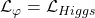We can study and write each ingredient/piece/sector of the EW theory separately:

1) Gauge sector.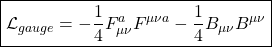The field strenth fields are defined as follows:The gauge fields are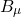the usual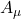electromagnetic field from classical relativistic theories and the non-abelian SU(2) gauge fields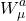where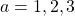.

There, g is the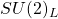coupling constant, andis the totally antisymmetric symbol or structure constants for the su(2) Lie algebra. The gauge group U(1) , classical electromagnetism, is abelian and thus, its structure constants are all zero. We also define an additional coupling constant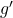for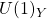, whose role and importance will be seen later.

The EW theory has three-point and four-point self-interactions due to the non-abelian nature of the weak interactions. The electromagnetic gauge fieldis afield related to the hypercharge operator. Then, the photon field has no self-interactions (unlike the non-abelian weak gauge fields). The gauge fieldsand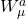mix in order to form the photon and the massive neutral Z field.

2) The scalar/Higgs sector.

The Higgs sector of the SM is provided by an extra piece in the lagrangian with the following form: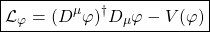where we define the doublet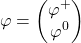It is a complex valued scalar field, generally named Higgs field. As it has two components, it is a doublet ( two component field) under the gauge groupwithcharge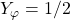. The gauge covariant derivative is defined to be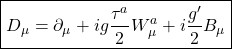whereare the Pauli matrices, the su(2) lie algebra generators in the fundamental representation of the gauge group SU(2). The constantis the coupling constant for the gauge group. In fact, the squared covariant derivative leads to three-point and four-point interactions between the gauge fields and the scalar fields in a natural way.

Finally, the mysterious extra piece in the Higgs sector is. It is called the scalar/Higgs potential. Some theoretical principles such as gauge invariance under the total EW groupand other physical conditions such as “renormalizability” ( finiteness of some physical quantities) restricts V to the following general form:The two terms in the Higgs potential are generally called: mass term (the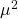part) and the self-coupling term ( the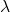part). The term describes a quartic self-interaction between the scalar fields. The mass term is also special. The spontaneous symmtry breaking (SSB) requires it to be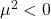, a phenomenon that is also known in the string community as a tachyon condensation. By the other hand, the existence of a stable ground state, i.e., the vacuum stability, implies that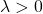, i.e., the self-coupling should be positive.

3) The fermionic sector.

The fermion piece in the EW theory can be written as the sum: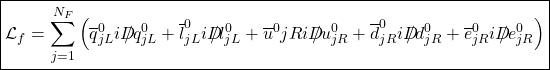In this lagrangian piece,runs fromto. In the SM, there are 3 families or generations. The notationis related to the left (right) chiral projectors, operators defined for every SM fermion as follows:The left-handed quark and lepton doublets are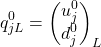The right-handed quark and lepton singles are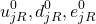The corresponding U(1) hypercharges are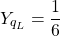and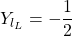and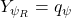Remark: The superscript 0 denotes weak eigenstates ( we used tilde in previous posts), i.e., they are fields transforming under some concreterepresentation. The weak eigenstates can be mixtures of mass eigenstates, and they are generally called flavor eigenstates. The color indices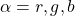must be understood in the case of quark fields and they were deleted for simplicity.

Finally, let me pount out that the covariant derivative written above acts on every quark and lepton field as well, and it provides gauge interactions between the W-bosons and the common photons ( B-fields in our notation). The different transformations of the L and R fields under the gauge group or the electroweak interaction explains why the SM is a “chiral theory”, i.e., it couples differently the left-handed fermions and the right-handed fermions. Moreover, observed neutrinos are left-handed only. There are no right-handed neutrinos in the SM, as we remarked before ( they are uncharged under the SM gauge group and they are not protected by any symmetry of the SM group). In fact, this chirality is the origin of the parity violation in the EW sector ( the V-A interaction was built in order to explain that parity violation from the experiments). And furthermore, this chiral symmetry also forbids the presence of any explicit bare mass terms for the fermions! This last observation was one of the reasons to invent the Higgs mechanism.

4) The Yukawa sector.

The final piece in the EW lagrangian is the Yukawa interaction termand where the matrices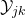describe the coupling between the single Higg doublet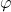and the different flavorsof quarks and leptons. Usually, these couplings between 3 fields are named Yukawa couplings.

In the minimal SM, we can choose representations of Higgs fields withand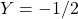for the Higgs scalars. This is the simplest election to generate masses for up-down quarks, electrons and likely to neutrino fields as well (although this last is less clear since the neutrino mass is very different from the other femions and gauge particles in the SM, a problem called the little hierarchy problem). The representation complex conjugate given by the fieldhas hypercharge, but it transforms as the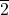rather than the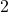. However, in the gauge group, therepresentation is related to theby a similatiry transformation, and thus we obtain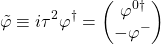and it transforms as a 2 with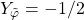.

In conclusion, all of the masses can be generated with a single Higgs doublet IF we useand.

Remark: The known group theoretical fact that the fundamental and its conjugate representation are equivalent does NOT generalize to higher unitary groups. It is a subtle point in modern group theory. Furthermore, in suspersymmetric extensions of the SM, SUSY forbids the use of a single Higgs doublet in both ways in the Lagrangian! Then, one must add a second Higgs doublet by hand ( or brute force, it you prefer the term). Similar statements apply to most theories (GUT’s, TOE’s) with an additionalgauge factor, i.e., to any theory with extra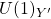groups ( e.g., strings, GUT’s groups) we are almost forced to introduce extra neutral Z bosons, the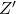fields. From the phenomenological viewpoint this is very interesting, since we have to search for resonances similar to the Z at “higher Z’-pole masses” (resonances) in order to discover evidences from these kind of theories.

This site uses Akismet to reduce spam. Learn how your comment data is processed.